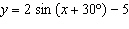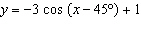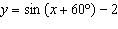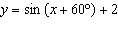Name:    5.3 Transformations

Multiple Choice
Identify the choice that best completes the statement or answers the question.

1.

What is the effect on a sine function when a changes from a positive value to a negative value?
 a. the amplitude becomes negative c. the function reflects in the x-axis b. the function reflects in the y-axis d. there is no effect on the function

2.

What are the horizontal and vertical translations of the function?
 a. 30° to the left and 5 units down c. 30° to the right and 5 units up b. 30° to the right and 5 units down d. 30° to the left and 5 units up

3.

What are the horizontal and vertical translations of the function?
 a. 45° to the left and 1 unit down c. 45° to the left and 1 unit up b. 45° to the right and 1 unit down d. 45° to the right and 1 unit up

4.

A sine function has a phase shift of 60° to the right and a vertical translation of 2 units down. What is a possible equation for this function?
 a.c.b.d.